• +91 9971497814
• info@interviewmaterial.com

# Chapter 4- Chemical Kinetics Interview Questions Answers

### Related Subjects

Question 1 :

For the reaction R → P,the concentration of a reactant changes from 0.03 M to 0.02 M in 25 minutes.Calculate the average rate of reaction using units of time both in minutes andseconds.

Answer 1 : Average rate of reaction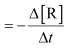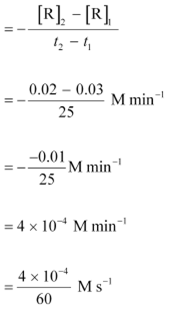= 6.67 × 10−6 M s−1

Question 2 :

In a reaction, 2A → Products, the concentration of A decreasesfrom 0.5 mol L−1 to0.4 mol L−1 in 10 minutes. Calculate the rate duringthis interval?

Answer 2 : Average rate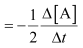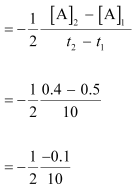= 0.005 mol L−1 min−1

= 5 × 10−3 M min−1

Question 3 : For a reaction, A + B → Product;the rate law is given by,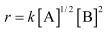. What is the order of the reaction?

Answer 3 : The order of the reaction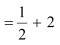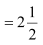= 2.5

Question 4 :

The conversion of molecules X toY follows second order kinetics. If concentration of X is increased to threetimes how will it affect the rate of formation of Y?

The reaction X → Y follows secondorder kinetics.

Therefore,the rate equation for this reaction will be:

Rate = k[X]2 (1)

Let [X] = a mol L−1,then equation (1) can be written as:

Rate1 = k .(a)2

ka2

If theconcentration of X is increased to three times, then [X] = 3a mol L−1

Now, therate equation will be:

Rate = k (3a)2

= 9(ka2)

Hence,the rate of formation will increase by 9 times.

Question 5 :

A first order reaction has a rateconstant 1.15 10−3 s−1. How long will 5 g of this reactant take toreduce to 3 g?

From the question, we can write down the following information:

Initialamount = 5 g

Finalconcentration = 3 g

Rate constant = 1.15 10−3 s−1

We know that for a 1st orderreaction,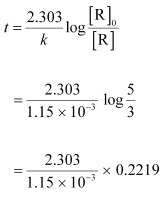= 444.38 s

= 444 s(approx)

Question 6 :

Time required to decompose SO2Cl2 to half of its initial amount is 60minutes. If the decomposition is a first order reaction, calculate the rateconstant of the reaction.

We know that for a 1st orderreaction,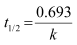It is given that t1/2 = 60 min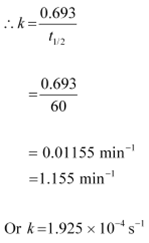Question 7 :

What will be the effect oftemperature on rate constant?

The rate constant of a reaction is nearly doubled with a 10° risein temperature. However, the exact dependence of the rate of a chemicalreaction on temperature is given by Arrhenius equation,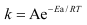Where,

A is the Arrhenius factoror the frequency factor

T is the temperature

R is the gas constant

Ea is the activation energy

Question 8 :

The rate of the chemical reactiondoubles for an increase of 10 K in absolute temperature from 298 K. Calculate Ea.

It is given that T1 = 298 K

T2 =(298 + 10) K

= 308 K

We alsoknow that the rate of the reaction doubles when temperature is increased by10°.

Therefore,let us take the value of k1 = k and that of k2 = 2k

Also, R = 8.314 J K−1 mol−1

Now,substituting these values in the equation: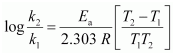We get: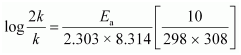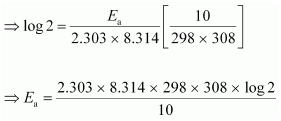= 52897.78 J mol−1

=52.9 kJ mol−1

Question 9 :

The activation energy for thereaction

2HI(g) → H2 + I2(g)

is 209.5 kJ mol−1 at 581K. Calculate the fraction ofmolecules of reactants having energy equal to or greater than activation energy?

the given case:

Ea = 209.5 kJ mol−1 = 209500 J mol−1

T = 581 K

R = 8.314 JK−1 mol−1

Now, the fraction of molecules of reactantshaving energy equal to or greater than activation energy is given as: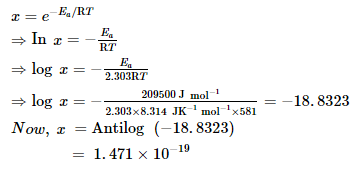Question 10 :

From the rate expression for thefollowing reactions, determine their order of reaction and the dimensions ofthe rate constants.

(ii) H2O(aq) + 3 I− (aq)+ 2 H+ → 2 H2O (l) +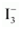Rate= k[H2O2][I]

(iii) CH3CHO(g)→ CH4(g)+ CO(g) Rate = [CH3CHO]3/2

(iv) C2H5Cl(g) → C2H4(g) + HCl(g) Rate = [C2H5Cl]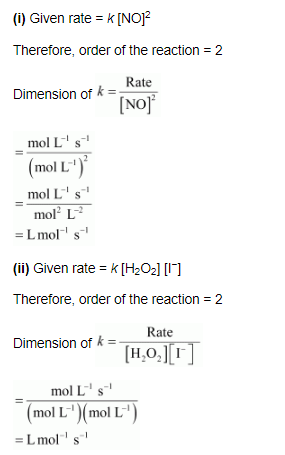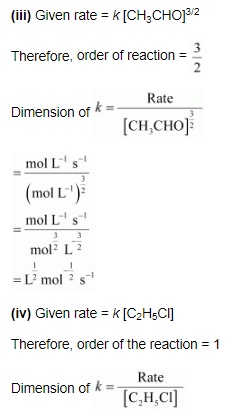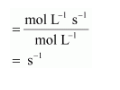Todays Deals### Chapter 4- Chemical Kinetics Contributorskrishan

Name:
Email:

# Latest News# 9000 interview questions in different categories